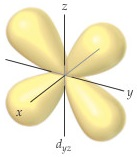# Problem: The accompanying drawing shows a contour plot for a dyz orbital. Consider the quantum numbers that could potentially correspond to this orbital. What is the smallest possible value of the principal quantum number, n?

###### Problem Details

The accompanying drawing shows a contour plot for a dyz orbital. Consider the quantum numbers that could potentially correspond to this orbital.What is the smallest possible value of the principal quantum number, n?